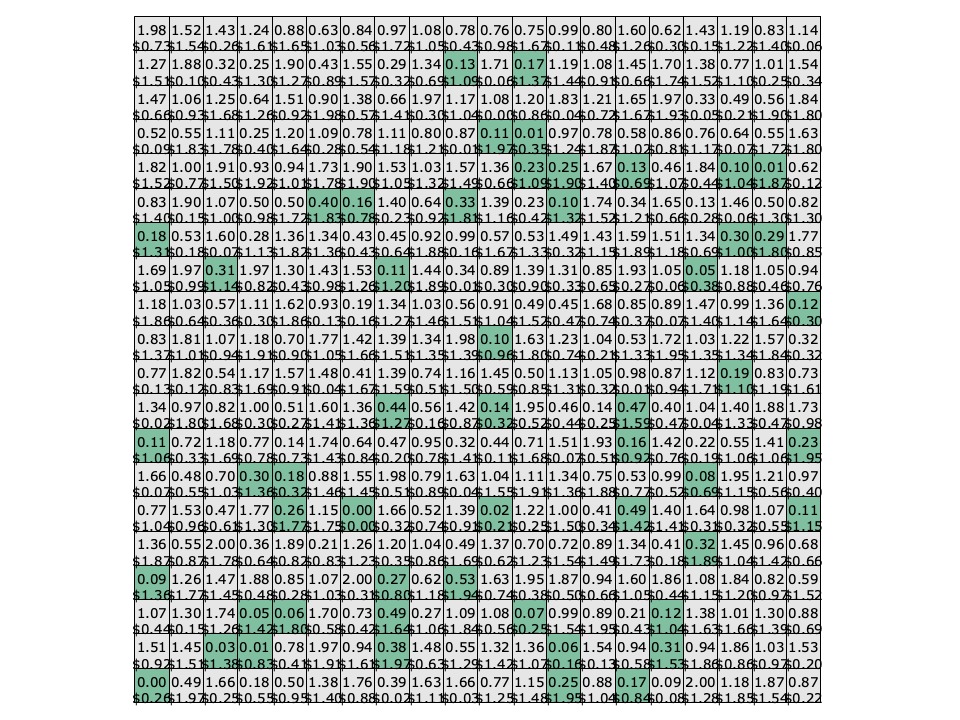# Genetic Algorithm Search Optimization (gasearch)

The gasearch object implements an evolutionary (genetic) algorithm search in liquid . The search uses a binary string of traits called a chromosome (see [section-optim-gasearch-chromosome] , below) to represent a potential solution. A population of chromosomes is generated and their appropriate fitnesses are calculated. With each evolution of the population the best chromosomes are retained and the worst are discarded; this process is known as selection . The population is restored by computing new potential solutions by splitting traits of the better chromosomes into a new member ( crossover ) as well as randomly flipping some of the bits in each chromosome ( mutation ).

## chromosome, solution representation

The chromosome object in liquid realizes a binary string of traits used in the gasearch object. A chromosome has a fixed number of traits as well as a fixed number of bits to represent each trait; however the number of bits representing each trait does not necessarily need to be the same for the chromosome. That is to say a chromosome may have a number of traits, each with a different number of bits representing them; however once a chromosome object is created, the number of bits representing each trait is not allowed to be changed.

Because of the many ways a chromosome can represent information liquid provides a number of methods for creating and initializing chromosomes.

• chromosome_create(*b,n) creates a chromosome with $$n$$ traits. The number of bits per trait are specified in the array $$\vec{b}$$ .
• chromosome_create_basic(n,b) creates a chromosome with $$n$$ traits and a constant $$b$$ bits for each trait.
• chromosome_create_clone(p) clones a chromosome from another one, including its representation of traits, the number of bits per trait, as well as the values of the traits themselves.
• chromosome_destroy(q) destroys a chromosome object, freeing all internally-allocated memory.

Furthermore, the value of all the chromosome's traits may be set with the appropriate init() method:

• chromosome_copy(q) copies an existing chromosomes' internal traits; all other internal parameters must be equal.
• chromosome_init(q,*v) initializes a chromosome's discrete trait values to the input array of unsigned int values $$\vec{v}$$ . The trait values are in the range $$[0,2^{n_k}-1]$$ where $$n$$ represents the number of bits in the $$k^{th}$$ trait.
• chromosome_initf(q,*v) initializes a chromosome's continuous trait values to the input array of float values $$\vec{v}$$ . The trait values are in the range $$[0,1]$$ and are represented by floating-point values. Because each trait has a discrete number of values (limited bit resolution), the value of the trait is quantized to its nearest representation.
• chromosome_init_random(q) initializes a chromosome's trait values to a random number.

The values of specific traits can be retrieved using the value() methods. They are useful for evaluating the fitness of the chromosome in the search algorithm's callback function.

• chromosome_value(q,k) returns the value of the $$k^{th}$$ trait (integer representation).
• chromosome_valuef(q,k) returns the value of the $$k^{th}$$ trait (floating-point representation).

Finally the methods for use in the gasearch algorithm are described:

• chromosome_mutate(q,k) flips the $$k^{th}$$ bit of the chromosome.
• chromosome_crossover(p1,p2,c,k) copies the first $$k$$ bits of the first parent $$p_1$$ and the remaining bits of the second parent $$p_2$$ to the child chromosome $$c_2$$ .

## Interface

Listed below is a description for the gasearch object in liquid .

• gasearch_create(*utility,*userdata,parent,min/max) creates a gasearch object, initialized on the specified parent chromosome. The user-defined utility function and userdata structures define the search, as well as the min/max flag which can be either LIQUID_OPTIM_MINIMIZE or LIQUID_OPTIM_MAXIMIZE .
• gasearch_destroy(q) destroys a gasearch object, freeing all internally-allocated memory.
• gasearch_print(q) prints the internal state of the gasearch object
• gasearch_set_mutation_rate(q,rate) sets the mutation rate
• gasearch_set_population_size(q,population,selection) sets both the population size as well as the selection size of the evolutionary algorithm
• gasearch_run(q,n,target_utility) runs multiple iterations of the search algorithm, stopping after either $$n$$ iterations or if the target utility is met.
• gasearch_evolve(q) steps through a single iteration of the search.
• gasearch_getopt(q,*chromosome,*u) produces the best chromosome over the coarse of the search evolution, as well as its utility.

## Example Code

An example of the gasearch interface is given below:


#include <liquid/liquid.h>

// user-defined utility callback function
float myutility(void * _userdata, chromosome _c)
{
// compute utility from chromosome
float u = 0.0f;
unsigned int i;
for (i=0; i<chromosome_get_num_traits(_c); i++)
u += chromosome_valuef(_c,i);
return u;
}

int main() {
unsigned int num_parameters = 8;        // dimensionality of search (minimum 1)
unsigned int num_iterations = 100;      // number of iterations to run
float target_utility = 0.01f;           // target utility

unsigned int bits_per_parameter = 16;   // chromosome parameter resolution
unsigned int population_size = 100;     // GA population size
float mutation_rate = 0.10f;            // GA mutation rate

// create prototype chromosome
chromosome prototype = chromosome_create_basic(num_parameters, bits_per_parameter);

// create gasearch object
&myutility,
NULL,
prototype,
LIQUID_OPTIM_MINIMIZE,
population_size,
mutation_rate);

// execute batch search
gasearch_run(ga, num_iterations, target_utility);

// execute search one iteration at a time
unsigned int i;
for (i=0; i<num_iterations; i++)
gasearch_evolve(ga);

// clean up objects
chromosome_destroy(prototype);
gasearch_destroy(ga);
}


Evolutionary algorithms are well-suited for discrete optimization problems, particularly where a large number of parameters only hold a few values. The classic example is the knapsack problem (constrained, non-linear) in which the selection of items with different weights and values must be chosen to maximize the total value without exceeding a prescribed weight capacity. An example of using the gasearch object in liquid to search over the solution space of the knapsack problem can be found in the examples directory as examples/gasearch_knapsack_example.c .

## ExampleFigure [fig-gasearch-example]. gasearch example (knapsack)